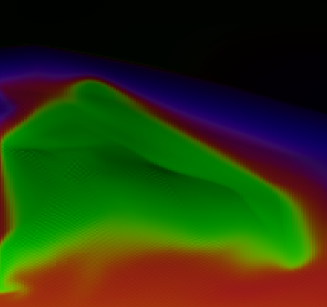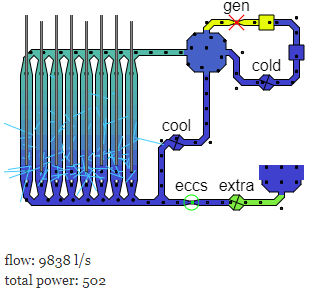﻿ Crystal Structure @Template

# Cubic Structures of Crystals

September 2021, Dr. Jennifer Martay

#### Challenges:

1. Pause each unit cell in the same position and take a screenshot (suggestioned position = looking directly at a front face).
2. Compare the sizes of the different types of unit cells.
3. Calculate how many atoms are inside each unit cell (note: only consider the parts INSIDE the unit cell).
4. Calculate the x, y, z coordinates of each atom in each unit cell, assuming the center point is <0,0,0>. To do this, you will need the following radius values:

BCC (Cr) r = 125 um
FCC (Al) r = 143 um
HCP (Zn) r = 133 um

#### Notes

1. The purple bits in HCP are overlapping from neighboring cells.

## Atmosphere

A simple demo of a simulation of an atmosphere. It looks quite cool, but there's not a lot you can do with it yet, and the physics isn't yet all that accurate.## Chernobyl Simulation

An attempt to simulate the normal running, and then accident of the Chernobyl nuclear reactor.

## Maths Exam Generator

Auto-generated maths exams, with and without answers. Set at A-level / end of high school / beginning of university.